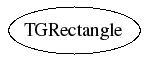library: libGui#include "TGDimension.h"

# TGRectangle

class description - header file - source file

## class TGRectangle### Function Members (Methods)

Display options:
Show inherited
Show non-public
public:
 TGRectangle() TGRectangle(const TGRectangle& r) TGRectangle(const TGPosition& p, const TGDimension& d) TGRectangle(Int_t rx, Int_t ry, UInt_t rw, UInt_t rh) virtual ~TGRectangle() Int_t Area() const static TClass* Class() Bool_t Contains(const TGPosition& p) const Bool_t Contains(Int_t px, Int_t py) const void Empty() Bool_t Intersects(const TGRectangle& r) const virtual TClass* IsA() const Bool_t IsEmpty() const TGPosition LeftTop() const void Merge(const TGRectangle& r) TGRectangle& operator=(const TGRectangle&) TGPosition RightBottom() const virtual void ShowMembers(TMemberInspector& insp, char* parent) TGDimension Size() const virtual void Streamer(TBuffer& b) void StreamerNVirtual(TBuffer& b)

### Data Members

public:
 Int_t fX x position Int_t fY y position UInt_t fW width UInt_t fH height

## Class Description

```
TGDimension, TGPosition, TGLongPosition, TGInsets and TGRectangle

Several small geometry classes that implement dimensions
(width and height), positions (x and y), insets and rectangles.
They are trivial and their members are public.

```

``` constructors
```
`{ Empty(); }`
TGRectangle(Int_t rx, Int_t ry, UInt_t rw, UInt_t rh)
`{ }`
TGRectangle(const TGPosition &p, const TGDimension &d)
`{ }`
TGRectangle(const TGRectangle &r)
`{ }`
virtual ~TGRectangle()
`{ }`
Bool_t Contains(Int_t px, Int_t py)
``` methods
```
Bool_t Contains(const TGPosition &p)
Bool_t Intersects(const TGRectangle &r)

`{ return (fW * fH); }`

`{ return TGDimension(fW, fH); }`

`{ return TGPosition(fX, fY); }`

`{ return TGPosition(fX + (Int_t) fW - 1, fY + (Int_t) fH - 1); }`
void Merge(const TGRectangle &r)
void Empty()
`{ fX = fY = 0; fW = fH = 0; }`

`{ return ((fW == 0) && (fH == 0)); }`